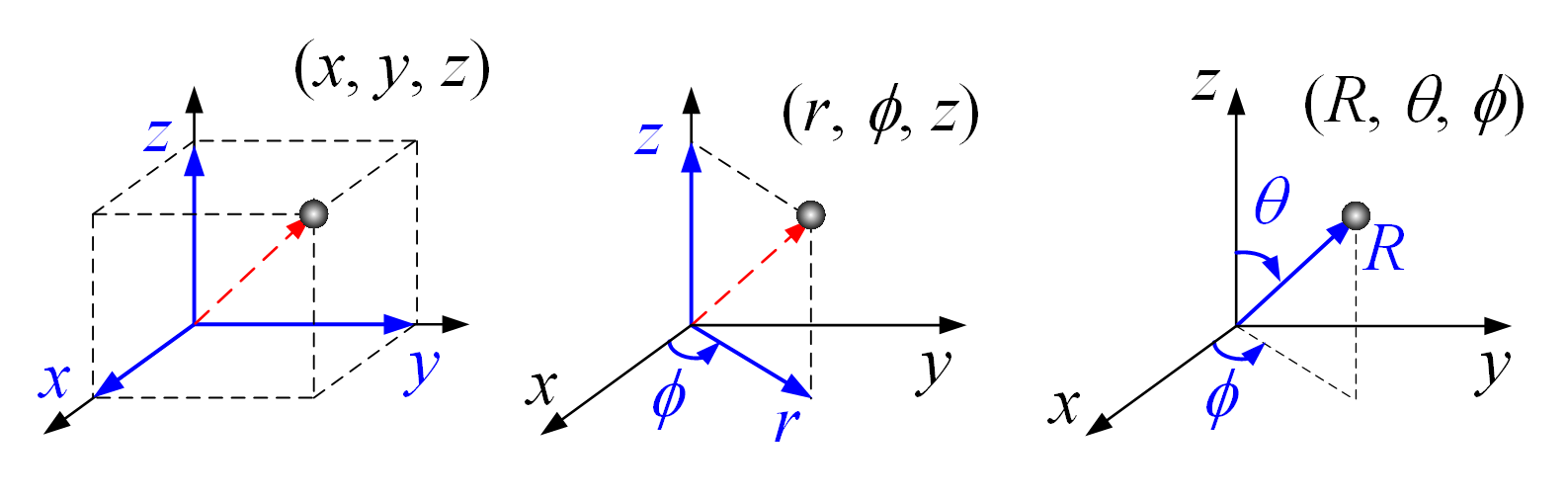Module 1.5 Volume Element of Various Coordinate Systems

Cartesian Coordinate system

Cylindrical Coordinate System

Spherical Coordinate System

 Cartesian Coordinates:    x = (m), y = (m), z = (m). Cylindrical Coordinates: r = (m), φ = o,     z = (m). Spherical Coordinates:    R = (m), θ = o,    φ = o.

Introduction:

Axis X：Red arrow
Axis Y：Green arrow
Axis Z：Blue arrow
1. Press the left mouse button and move the mouse cursor to change the viewpoint, or you can click the standard view button in the upper right corner.
2. Press the right mouse button and move the graph with your mouse.

Parameters:Cartesian coordinates     Cylindrical coordinates      Spherical coordinates